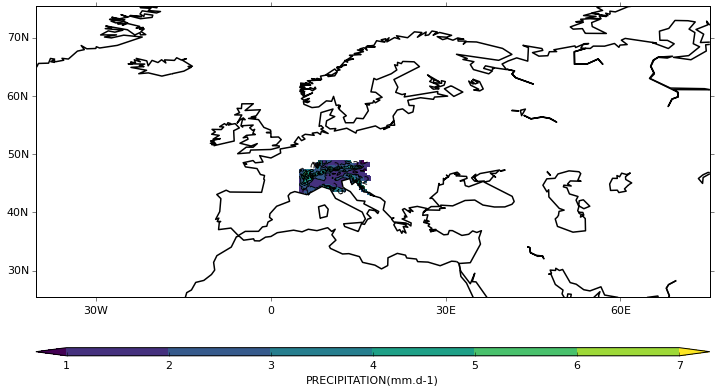In this example we work through the following steps:

1. Import the cf package.
2. Read a field from disk and find a summary of its contents.
3. Read in a second field and find a summary of its contents.
4. Regrid the first field to the grid of the second bilinearly and find a summary of the resulting field's contents.
5. Import the cf-plot package.
6. Plot the results.

1. Import the cf package.

In :
import cf


2. Read a field from disk and find a summary of its contents.

In :
f = cf.read('EURO4M-APGD_DJF_precip_means.nc')
f

Out:
<CF Field: long_name:PRECIPITATION(long_name:time(1), projection y coordinate(138), projection x coordinate(243)) mm.d-1>
In :
print f

long_name:PRECIPITATION field summary
-------------------------------------
Data           : long_name:PRECIPITATION(long_name:time(1), projection y coordinate(138), projection x coordinate(243)) mm.d-1
Cell methods   : long_name:time: mean
Axes           : long_name:time(1) = [1996-07-15 12:00:00] standard
: projection y coordinate(138) = [2210000.0, ..., 2895000.0] meters
: projection x coordinate(243) = [3675000.0, ..., 4885000.0] meters
Aux coords     : longitude(projection y coordinate(138), projection x coordinate(243)) = [[2.12521505356, ..., 17.7066478729]] degrees_east
: latitude(projection y coordinate(138), projection x coordinate(243)) = [[42.6916656494, ..., 48.9023704529]] degrees_north
Coord refs     : <CF CoordinateReference: lambert_azimuthal_equal_area>



3. Read in a second field and find a summary of its contents.

In :
g = cf.read('E-OBS_DJF_precip_means.nc')
g

Out:
<CF Field: thickness_of_rainfall_amount(time(1), latitude(201), longitude(464)) mm>
In :
print g

thickness_of_rainfall_amount field summary
------------------------------------------
Data           : thickness_of_rainfall_amount(time(1), latitude(201), longitude(464)) mm
Cell methods   : time: mean
Axes           : time(1) = [1996-07-15 12:00:00]
: latitude(201) = [25.375, ..., 75.375] degrees_north
: longitude(464) = [-40.375, ..., 75.375] degrees_east



4. Regrid the first field to the grid of the second bilinearly and find a summary of the resulting field's contents.

In :
f.dim('projection x coordinate').axis = 'X'
f.dim('projection y coordinate').axis = 'Y'
h = f.regrids(g, method='bilinear')
h

Out:
<CF Field: long_name:PRECIPITATION(long_name:time(1), latitude(201), longitude(464)) mm.d-1>
In :
print h

long_name:PRECIPITATION field summary
-------------------------------------
Data           : long_name:PRECIPITATION(long_name:time(1), latitude(201), longitude(464)) mm.d-1
Cell methods   : long_name:time: mean
Axes           : long_name:time(1) = [1996-07-15 12:00:00] standard
: latitude(201) = [25.375, ..., 75.375] degrees_north
: longitude(464) = [-40.375, ..., 75.375] degrees_east



5. Import the cfplot package.

In :
%matplotlib inline

import cfplot as cfp


6. Plot the results.

In :
cfp.con(h)

/home/charles/anaconda/lib/python2.7/site-packages/matplotlib/collections.py:650: FutureWarning: elementwise comparison failed; returning scalar instead, but in the future will perform elementwise comparison
if self._edgecolors_original != str('face'):
/home/charles/anaconda/lib/python2.7/site-packages/matplotlib/collections.py:590: FutureWarning: elementwise comparison failed; returning scalar instead, but in the future will perform elementwise comparison
if self._edgecolors == str('face'):
/home/charles/anaconda/lib/python2.7/site-packages/matplotlib/text.py:52: UnicodeWarning: Unicode equal comparison failed to convert both arguments to Unicode - interpreting them as being unequal
if rotation in ('horizontal', None):
/home/charles/anaconda/lib/python2.7/site-packages/matplotlib/text.py:54: UnicodeWarning: Unicode equal comparison failed to convert both arguments to Unicode - interpreting them as being unequal
elif rotation == 'vertical':